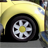# New to Qlik Sense

If you’re new to Qlik Sense, start with this Discussion Board and get up-to-speed quickly.

Announcements
Customer & Partners, DEC. 9, 11 AM ET: Qlik Product & Strategy Roadmap Session: Data Analytics REGISTER NOW
cancel
Showing results for
Did you mean:Specialist

## Qlik sense Set analysis

In my dataset, the yearField is from 2010 to 2017. I would like to get the sum of most recent 10 year with Qlik sense Set analysis in KPI,

1: when I use:

sum( {\$< yearField = {"> 2007 "}  > } yearField ) ,

will get 20125 and it works.

2: but I need make the expression dynamic (2018, 2019, etc in the future)

3: When I used a Qlik function in Set analysis:

sum( {\$< yearField = {">(=( Year( Today()) -10 )) "}  > } yearField )

will get 0 but does not work any more.

4: I also tried with variable.

define a variable, Year( Today()) - 10  (variable name: MostRecent10Years)

when I used the variable

Sum({ <yearField = { '\$( > \$(MostRecent10Years) )' }>}  yearField )

will get 0 but does not work either.

Any suggestion is appreciated!

1 Solution

Accepted SolutionsContributor III

use step 4, like this

try:

define a variable,   "=Year( Today()) - 10 " (variable name: MostRecent10Years)

use variable:

Sum({ <yearField = { '>\$(MostRecent10Years)' }>}  yearField )

but to do dinamic i recomend u create variable like this:

"=MAX(YEARFIELD)-10"

if this works plz mark this!!

thx

REGARDS!

10 RepliesSpecialist

Define variable MostRecent10Years as

Num(Year(Today())-10)Contributor III

use step 4, like this

try:

define a variable,   "=Year( Today()) - 10 " (variable name: MostRecent10Years)

use variable:

Sum({ <yearField = { '>\$(MostRecent10Years)' }>}  yearField )

but to do dinamic i recomend u create variable like this:

"=MAX(YEARFIELD)-10"

if this works plz mark this!!

thx

REGARDS!Specialist

May be this

sum( {\$< yearField = {">=\$(=Year(AddYears(Today(),-10)))} <=\$(=Year(Today())) > } yearField ) ,Specialist
Author

Thanks all!

1:

Define variable MostRecent10Years as

Num(Year(Today())-10)

Still get 0

2:

define a variable,   "=Year( Today()) - 10 " (variable name: MostRecent10Years)

use variable:

Sum({ <yearField = { '>\$(MostRecent10Years)' }>}  yearField )

still get 0

3:

create variable like this:

"=MAX(YEARFIELD)-10"

still get 0

4:

sum(

{< yearField = {'>=\$(=Year(AddYears(Today(),-10)))} <=\$(=Year(Today()))' > }

yearField )

return nothing.

Any suggestion is appreciated!Contributor III

u remove the double quotes right?

ur yearField  have something ?

and now i see, u are make sum of the yearField? u need take another field to sum... no?Specialist
Author

I put the following in the qlik variable editor:

MAX(yearField)-10

thanks!Contributor III

but u need use sinal of iguals (=) for this worksSpecialist

sorry small error i missed "}

try now

sum( {\$< yearField = {">=\$(=Year(AddYears(Today(),-10)))} <=\$(=Year(Today())) "}> } yearField )Specialist

i think this should work

sum( {\$< yearField = {">\$(=Year(Today() -10)) "}  > } yearField )Tags
Community Browser